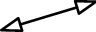# Table 1 Elemental representation of atlas

Atlas elements Physical significance Helical vector typeForce constraints Line vector
$${\ }^{r} = ({\mathbf{S}} ; { }{\mathbf{S}}_{0} )$$Couple constraint Couple vector
$${\ }^{r} = (0 ; { }{\mathbf{S}})$$The rotating DOF Line vector
$${\} = ({\mathbf{S}} ; { }{\mathbf{S}}_{0} )$$The moving DOF  Couple vector
$${\ } = (0 ; { }{\mathbf{S}})$$
1. In the table, $${\}^{r}$$ and $${\ }$$ are the spiral expressions of constraints and movements